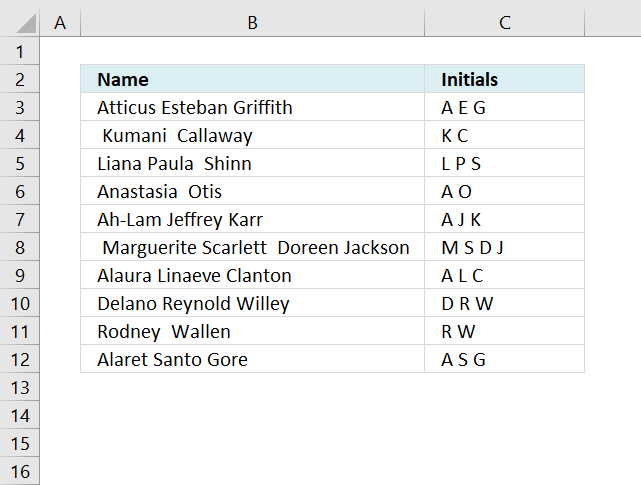Author: Oscar Cronquist Article last updated on December 06, 2018The array formula in cell C3 extracts the first character from first, middle and last name. The formula works fine with more or less names, up to 1000 characters. You can easily edit the formula if you need more letters.

Array formula in cell C3:

=TRIM(TEXTJOIN("", TRUE, IF(MID(" "&TRIM(B3), ROW(\$B\$1:\$B\$1000), 1)=" ", " "&MID(TRIM(B3), ROW(\$B\$1:\$B\$1000), 1), "")))

To enter an array formula, type the formula in a cell then press and hold CTRL + SHIFT simultaneously, now press Enter once. Release all keys.

The formula bar now shows the formula with a beginning and ending curly bracket telling you that you entered the formula successfully. Don't enter the curly brackets yourself.

### Explaining formula in cell C3

#### Step 1 - Split name into characters

The MID function returns characters from a text string based on a start number and the number of characters. The ROW function creates an array in this case that we need to split the text string into characters. The TRIM function removes leading and trailing spaces.

MID(" "&TRIM(B3), ROW(\$B\$1:\$B\$1000), 1)

becomes

MID(" "&TRIM(B3), {1;2;3;4;5;6;7;8;9; ... ; 1000}, 1)

becomes

MID(" Atticus Esteban Griffith", {1;2;3;4;5;6;7;8;9; ... ; 1000}, 1)

and returns

{" ";"A";"t";"t";"i";"c";"u";"s";" "; "E";"s";"t";"e";"b"; "a";"n";" ";"G";"r"; "i";"f";"f";"i"; "t";"h";""; ... ;""}.

#### Step 2 - Check if a character is a space (blank)

The IF function replaces space characters with the next character.

IF(MID(" "&TRIM(B3), ROW(\$B\$1:\$B\$1000), 1)=" ", " "&MID(TRIM(B3), ROW(\$B\$1:\$B\$1000), 1), "")

becomes

IF({" ";"A";"t";"t";"i"; "c";"u";"s";" ";"E";"s"; "t";"e";"b";"a"; "n";" ";"G";"r"; "i";"f";"f";"i"; "t";"h";""; ... ;""}=" ", " "&MID(TRIM(B3), ROW(\$B\$1:\$B\$1000), 1), "")

becomes

IF({TRUE; FALSE; FALSE; FALSE; FALSE; FALSE; FALSE; FALSE; TRUE; FALSE; FALSE; FALSE; FALSE; FALSE; FALSE; FALSE; TRUE; FALSE; FALSE; FALSE; FALSE; FALSE; FALSE; FALSE; FALSE; FALSE; FALSE}, " "&MID(TRIM(B3), ROW(\$B\$1:\$B\$1000), 1), "")

becomes

IF({TRUE; FALSE; FALSE; FALSE; FALSE; FALSE; FALSE; FALSE; TRUE; FALSE; FALSE; FALSE; FALSE; FALSE; FALSE; FALSE; TRUE; FALSE; FALSE; FALSE; FALSE; FALSE; FALSE; FALSE; FALSE; FALSE; FALSE}, {" A"; " t"; " t"; " i"; " c"; " u"; " s"; " "; " E"; " s"; " t"; " e"; " b"; " a"; " n"; " "; " G"; " r"; " i"; " f"; " f"; " i"; " t"; " h"; ... ; " "}, "")

and returns

{" A";"";"";"";"";"";"";"";" E";"";"";"";"";"";"";"";" G";"";"";"";"";"";"";"";"";""; ... ; ""}

#### Step 3 - Concatenate characters

The TEXTJOIN function concatenates characters in an array and in this case ignoring empty array values.

TRIM(TEXTJOIN("", TRUE, IF(MID(" "&TRIM(B3), ROW(\$B\$1:\$B\$1000), 1)=" ", " "&MID(TRIM(B3), ROW(\$B\$1:\$B\$1000), 1), "")))

becomes

TRIM(TEXTJOIN("", TRUE, {" A";"";"";"";"";"";"";"";" E";"";"";"";"";"";"";"";" G";"";"";"";"";"";"";"";"";""; ... ; ""}))

becomes

TRIM(" A E G")

The TRIM function removes leading and trailing spaces.

TRIM(" A E G") returns "A E G" in cell C3.

Initialsv3.xlsx